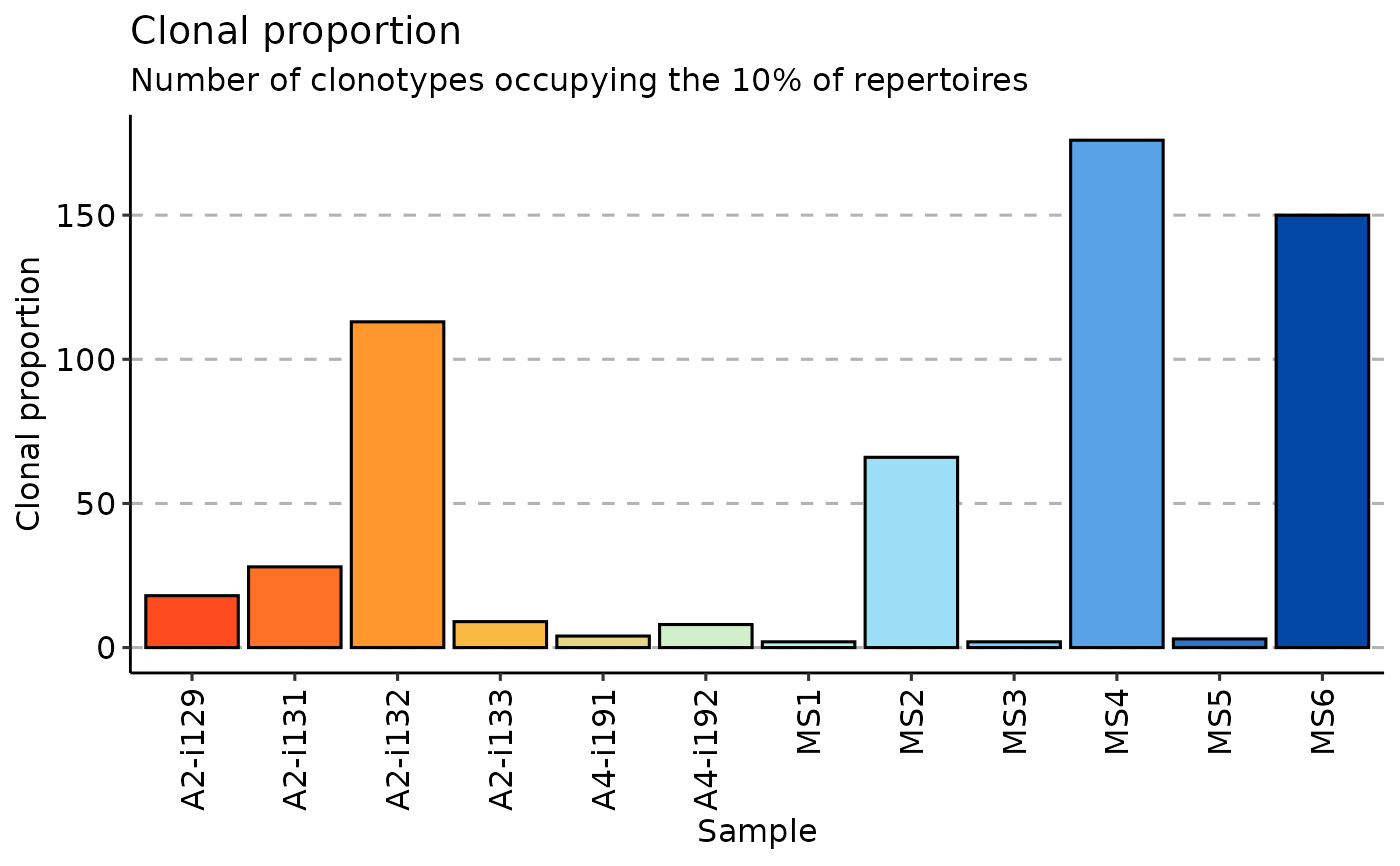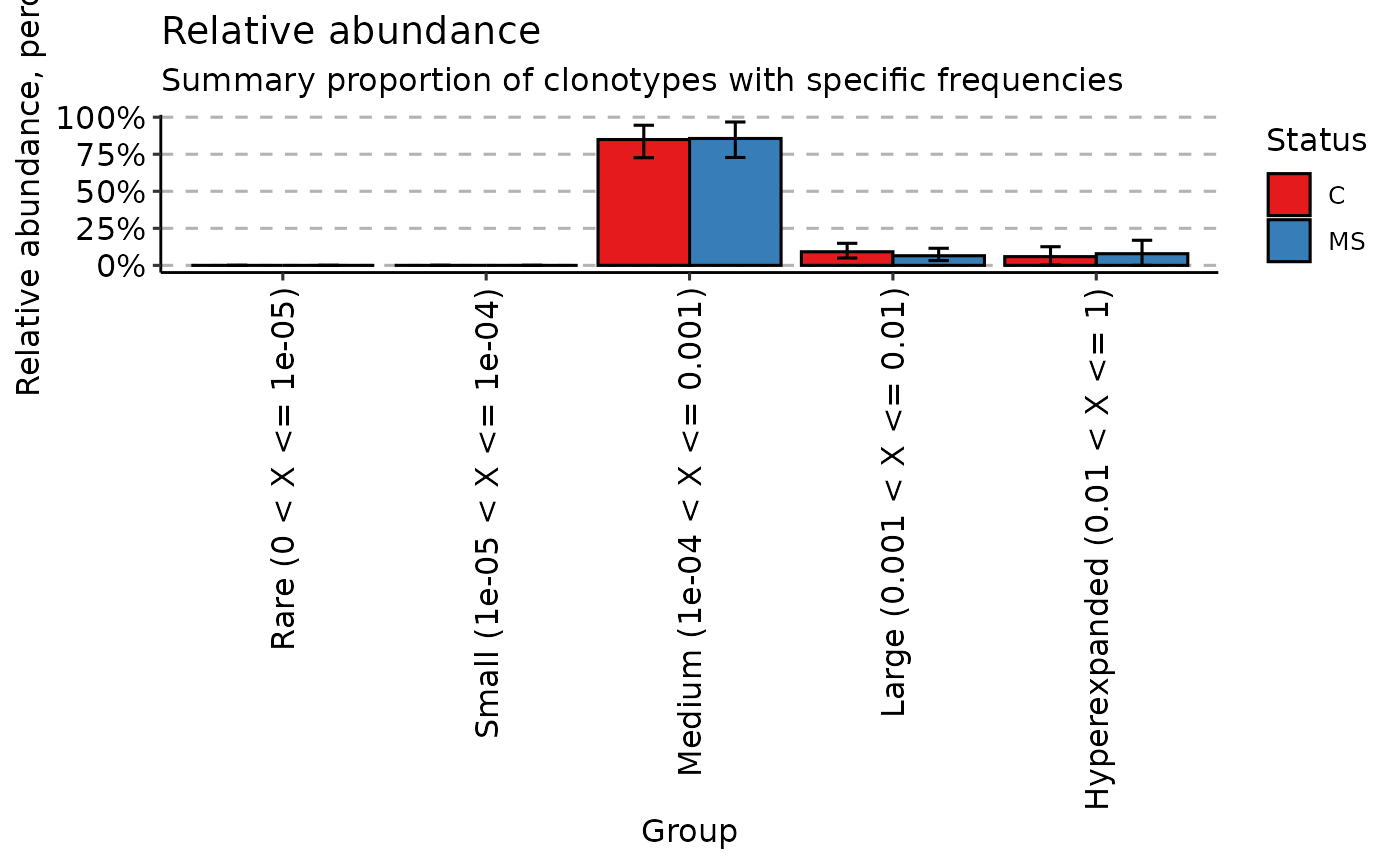An utility function to visualise the output from repClonality.

# S3 method for immunr_clonal_prop
vis(
.data,
.by = NA,
.meta = NA,
.errorbars = c(0.025, 0.975),
.errorbars.off = FALSE,
.points = TRUE,
.test = TRUE,
.signif.label.size = 3.5,
...
)

## Arguments

.data

Output from repClonality.

.by

Pass NA if you want to plot samples without grouping.

You can pass a character vector with one or several column names from ".meta" to group your data before plotting. In this case you should provide ".meta".

You can pass a character vector that exactly matches the number of samples in your data, each value should correspond to a sample's property. It will be used to group data based on the values provided. Note that in this case you should pass NA to ".meta".

.meta

A metadata object. An R dataframe with sample names and their properties, such as age, serostatus or hla.

.errorbars

A numeric vector of length two with quantiles for error bars on sectors. Disabled if ".errorbars.off" is TRUE.

.errorbars.off

If TRUE then plot CI bars for distances between each group. Disabled if no group passed to the ".by" argument.

.points

A logical value defining whether points will be visualised or not.

.test

A logical vector whether statistical tests should be applied. See "Details" for more information.

.signif.label.size

An integer value defining the size of text for p-value.

...

Not used here.

## Value

A ggplot2 object.

## Details

If data is grouped, then statistical tests for comparing means of groups will be performed, unless .test = FALSE is supplied. In case there are only two groups, the Wilcoxon rank sum test (https://en.wikipedia.org/wiki/Wilcoxon_signed-rank_test) is performed (R function wilcox.test with an argument exact = FALSE) for testing if there is a difference in mean rank values between two groups. In case there more than two groups, the Kruskal-Wallis test (https://en.wikipedia.org/wiki/Kruskal A significant Kruskal-Wallis test indicates that at least one sample stochastically dominates one other sample. Adjusted for multiple comparisons P-values are plotted on the top of groups. P-value adjusting is done using the Holm method (https://en.wikipedia.org/wiki/Holm You can execute the command ?p.adjust in the R console to see more.

data(immdata)
clp <- repClonality(immdata$data, "clonal.prop") vis(clp)hom <- repClonality(immdata$data, "homeo")